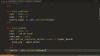In Progress
Unit 1, Lesson 1
In Progress

# Testing with Coat Hangers

What happens when you try to TDD new functionality deep inside a private method? The resulting tests can feel terribly awkward and brittle.Video transcript & code

So, here we are, making a sandwich using a pair of coat hangers. And it’s not going very well.

Hmmm… maybe I should back up.

In a previous episode, we used test-driven design to implement a rudimentary reverse-polish notation calculator.

And a `case`-based implementation.

Because our functionality was covered by tests, we were able to safely extract the operator cases into a private method called `lookup_binary_operator`.

And since the public method we refactored from was tested, so was the private extracted method.

But what if we now want to add a new operator to our calculator?

Say we want to add a `rand` operator that interprets the last two numbers in the stack as inclusive lower and upper bounds, and generates a random number between those two bounds.

How might we drive this new functionality with tests?

Today we’re going to start by doing this in a way that I wouldn’t recommend. And then we’ll talk a little about why this approach is problematic. Let’s get started.

We instantiate a `Calc` instance, and an example expression that makes use of the `rand` operator.

Now we need to test that this expression generates a random number.

To do that, we decide that we’ll be using Ruby’s `rand` method under the hood.

So we need to mock out the system `rand` method for this `Calc` object.

Instead of using a mock-object library, we’ll just mock it out in pure Ruby.

First, we prepare a probe variable to capture the upper-bound argument passed to the system `rand` method.

Then we override the system `rand` method on the `calc` instance.

inside, we capture the value passed to `rand` into our probe variable, for later verification.

And we return a stubbed random result of `3`.

With all that set up, we tell our object under test to calculate the expression.

Our test expression says to generate a random number between 10 and 20, inclusive. We expect our implementation to adjust this interval to be zero-based, and add one for inclusiveness. So we assert that the argument passed to `rand` was 11.

We then expect the implementation to adjust the result of `rand` back up to the original 10-20 range, so we assert that the result of the calculation is 13.

``````  def test_random
calc = Calc.new
expr = "10 20 rand"
actual_rand_upper_bound = :unset
calc.define_singleton_method :rand do |upper_bound|
actual_rand_upper_bound = upper_bound
3
end
result = calc.calculate(expr)
assert_equal 11, actual_rand_upper_bound
assert_equal 13, result
end
``````

Whew. That was an elaborate test.

Which of course fails at the moment.

To implement it, we can add a new entry in our operator table. We’ll map it to another new private method called `apply_rand`.

We write that method to do all the math gymnastics that we just specified in the test, in order to generate a random number in the requested range.

``````require "minitest/autorun"

class TestCalc < MiniTest::Test
calc = Calc.new
expr = "3 2 +"
assert_equal 5, calc.calculate(expr)
end

def test_subtract
calc = Calc.new
expr = "10 7 -"
assert_equal 3, calc.calculate(expr)
end

def test_random
calc = Calc.new
expr = "10 20 rand"
actual_rand_upper_bound = :unset
calc.define_singleton_method :rand do |upper_bound|
actual_rand_upper_bound = upper_bound
3
end
result = calc.calculate(expr)
assert_equal 11, actual_rand_upper_bound
assert_equal 13, result
end
end

class Calc

def initialize
@stack = []
end

def calculate(expression)
expression.split.each do |token|
case token
when /^\d+\$/
stack.push(Integer(token))
when "+", "-", "rand"
right = stack.pop
left = stack.pop
op = lookup_binary_operator(token)
stack.push(op.call(left, right))
else
fail "Unexpected token: '#{token}'"
end
end
stack.last
end

private

def lookup_binary_operator(token)
{
"+" => ->(left, right) { left + right },
"-" => ->(left, right) { left - right },
"rand" => method(:apply_rand),
}.fetch(token)
end

def apply_rand(lower_bound, upper_bound)
rand(upper_bound - lower_bound + 1) + lower_bound
end
end

``````

Finally, we add “rand” to the list of allowable binary operators.

The tests now pass again.

But oh, this test. This test does not make us happy.

We want tests to express the intent of the method being tested, not the implementation. But this test is tied inextricably to a very specific implementation of random number generation.

And in order to test that very specific implementation, it mocks out a system call.

This is one of the big no-nos for mocking and stubbing. As the inventors of mock-object testing put it, we should “Only Mock What We Own”.

The root of the problem here is that we‘re “testing at a distance”. We’re writing tests with the assumption that we’re going to add some new functionality to an existing private method. In order to test-drive the addition at a remove, we have to go through some awkward contortions to set up the conditions for the test. It’s kind of like trying to make a sandwich… using only a pair of coathangers.

This problem of testing-at-a-distance can tempt us to test the private methods directly, or make them public in order to apply tests to them. But that’s not the only option. We’ll talk about an alternative in an upcoming episode. Happy hacking!